Main Content

## Visualize Effects of Frequency-Selective Fading

### FSK Modulation in Fading Channel

Pass an FSK signal through a Rayleigh multipath fading channel. Change the signal bandwidth to observe the impact of the fading channel on the FSK spectrum.

FSK Modulation in Flat Fading

Set modulation order to 4, the modulated symbol rate to 45 bps, and the frequency separation to 200 Hz.

```M = 4; % Modulation order symbolRate = 45; % Symbol rate (bps) freqSep = 200; % Frequency separation (Hz)```

Calculate the samples per symbol parameter, `sampPerSym`, as a function of the modulation order, frequency separation, and symbol rate. To avoid output signal aliasing, the product of `sampPerSym` and `symbolRate` must be greater than the product of `M` and `freqSep`. Calculate the sample rate of the FSK output signal.

```sampPerSym = ceil(M*freqSep/symbolRate); fsamp = sampPerSym*symbolRate;```

Create an FSK modulator.

```fskMod = comm.FSKModulator(M, ... 'FrequencySeparation',freqSep, ... 'SamplesPerSymbol',sampPerSym, ... 'SymbolRate',symbolRate);```

Set the path delays and average path gains for the fading channel.

```pathDelays = [0 3 10]*1e-6; % Discrete delays of three-path channel (s) avgPathGains = [0 -3 -6]; % Average path gains (dB)```

By convention, the delay of the first path is typically set to zero. For subsequent paths, a 1 microsecond delay corresponds to a 300 m difference in path length. The path delays and path gains specify the average delay profile of the channel.

Create a Rayleigh channel using the defined parameters. Set the `Visualization` property to display the impulse and frequency responses.

```channel = comm.RayleighChannel(... 'SampleRate',fsamp, ... 'PathDelays',pathDelays, ... 'AveragePathGains',avgPathGains, ... 'MaximumDopplerShift',0.01, ... 'Visualization','Impulse and frequency responses', ... 'SamplesToDisplay','10%');```

Generate random data symbols and apply FSK modulation.

```data = randi([0 3],2000,1); modSig = fskMod(data);```

Plot the spectrum of the FSK modulated signal.

```spectrum = dsp.SpectrumAnalyzer('SampleRate',fsamp); spectrum(modSig)```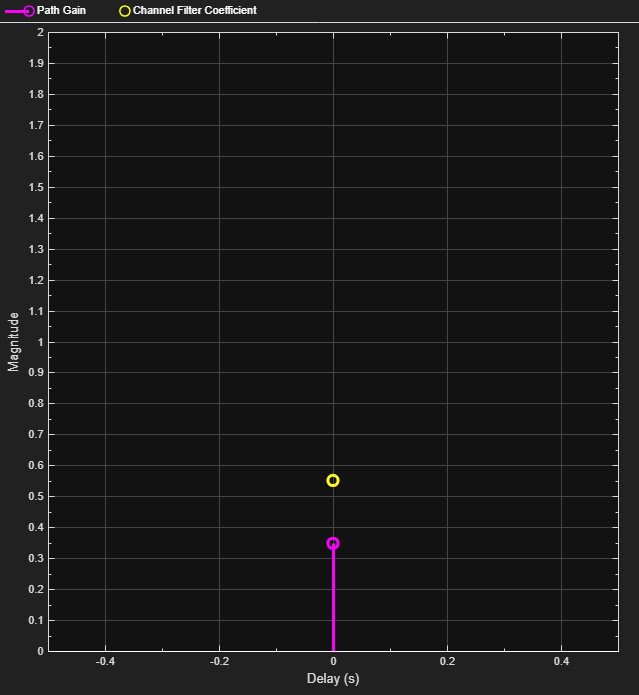The modulated signal is composed of four tones each having approximately 20 dBm peak power separated by 200 Hz.

Pass the signal through the Rayleigh fading channel and apply AWGN having a 25 dB signal-to-noise ratio.

```snrdB = 25; rxSig = awgn(channel(modSig),snrdB);```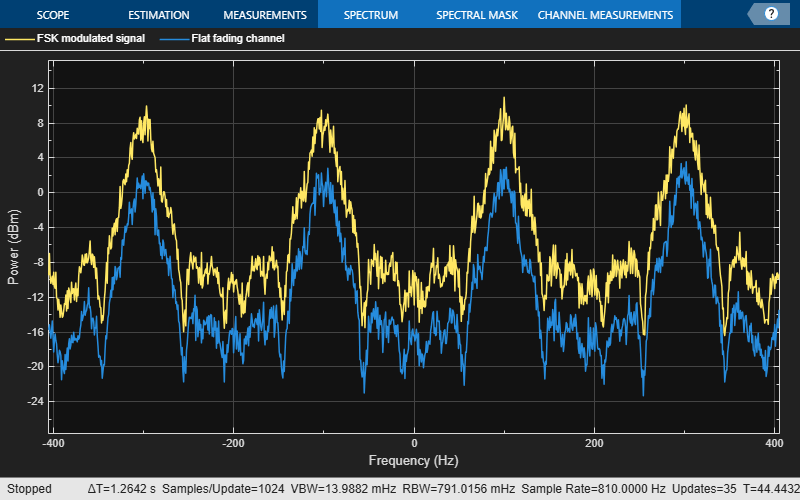The impulse and frequency responses show that the channel behaves as though it were flat. This is because the signal bandwidth, 800 Hz, is much smaller than the coherence bandwidth, 50 kHz.

Plot the received signal spectrum.

`spectrum(rxSig)`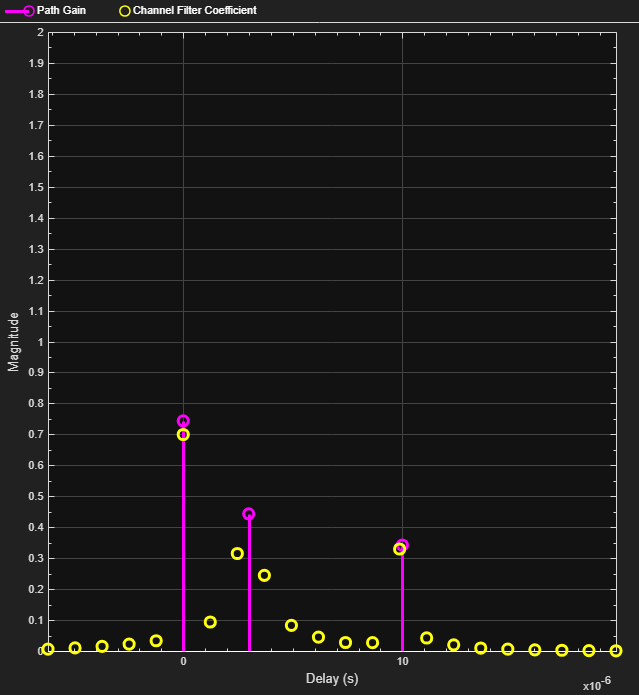The four tones comprising the FSK signal maintain the same frequency separation and peak power levels relative to each other. The absolute peak power levels have decreased due to the fading channel.

FSK Modulation in Frequency-Selective Fading

Increase the symbol rate to 45 kbps and the frequency separation to 200 kHz. Calculate the new samples per symbol and sample rate parameters.

```symbolRate = 45e3; freqSep = 200e3; sampPerSym = ceil(M*freqSep/symbolRate); fsamp = sampPerSym*symbolRate;```

Update the FSK modulator properties.

```release(fskMod) fskMod.SymbolRate = symbolRate; fskMod.FrequencySeparation = freqSep;```

Update the spectrum analyzer sample rate property, `sa.SampleRate`. Apply FSK modulation and plot the resulting spectrum.

```release(spectrum) spectrum.SampleRate = sampPerSym*symbolRate; modSig = fskMod(data); spectrum(modSig)```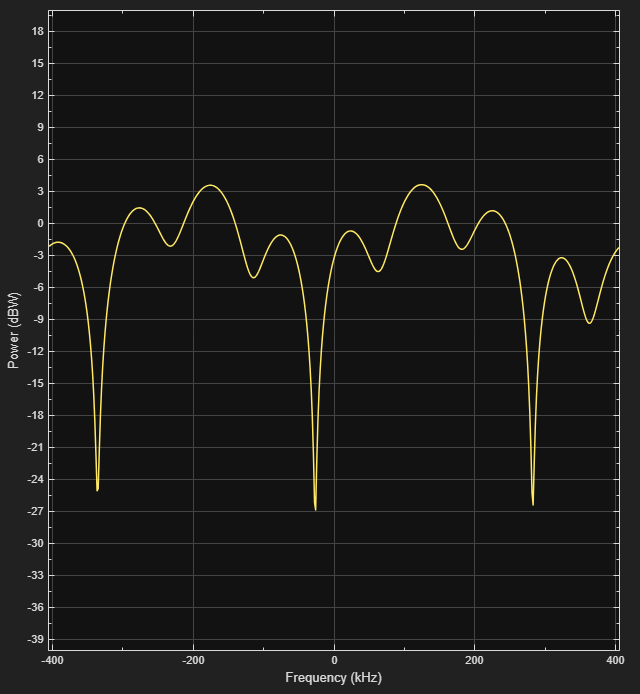The spectrum has the same shape as in the flat-fading case but the four tones are now separated by 200 kHz.

Update the channel sample rate property. Pass the signal through the Rayleigh fading channel and apply AWGN.

```release(channel) channel.SampleRate = fsamp; rxSig = awgn(channel(modSig),25);```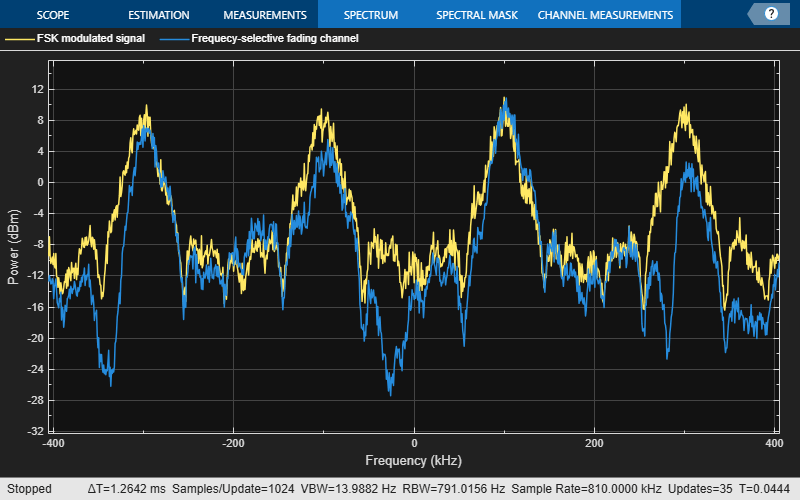The impulse and frequency responses show that the multipath fading is frequency selective.

Plot the received signal spectrum.

`spectrum(rxSig)`There are still four identifiable tones but their relative peak power levels differ due to the frequency-selective fading. The signal bandwidth, 800 kHz, is larger than the coherence bandwidth, 50 kHz.

### QPSK Modulation in Fading Channel

Pass a QPSK signal through a Rayleigh multipath fading channel. Change the signal bandwidth to observe the impact of the fading channel on the QPSK constellation.

QPSK Modulation in Flat Fading

Set the symbol rate parameter to 500 bps.

```symbolRate = 500; ```

Generate random data symbols and apply QPSK modulation.

```data = randi([0 3],10000,1); modSig = pskmod(data,4,pi/4,'gray'); ```

Set the path delays and average path gains for the fading channel.

```pathDelays = [0 3 10]*1e-6; % Discrete delays of three-path channel (s) avgPathGains = [0 -3 -6]; % Average path gains (dB) ```

By convention, the delay of the first path is typically set to zero. For subsequent paths, a 1 microsecond delay corresponds to a 300 meter difference in path length. The path delays and path gains specify the average delay profile of the channel.

Create a Rayleigh channel using the defined parameters. Set the `Visualization` property to display the impulse and frequency responses.

```fsamp = symbolRate; channel = comm.RayleighChannel(... 'SampleRate',fsamp, ... 'PathDelays',pathDelays, ... 'AveragePathGains',avgPathGains, ... 'MaximumDopplerShift',0.01, ... 'Visualization','Impulse and frequency responses'); ```

Pass the signal through the Rayleigh channel and apply AWGN.

```rxSig = awgn(channel(modSig),25); ```The impulse and frequency responses show that the channel behaves as though it were flat. This is because the signal bandwidth, 500 Hz, is much smaller than the coherence bandwidth, 50 kHz. Alternatively, the delay span of the channel (10 microseconds) is much smaller than the QPSK symbol period (2 milliseconds) so the resultant bandlimited impulse response is approximately flat.

Plot the constellation.

```constDiagram = comm.ConstellationDiagram; constDiagram(rxSig) ```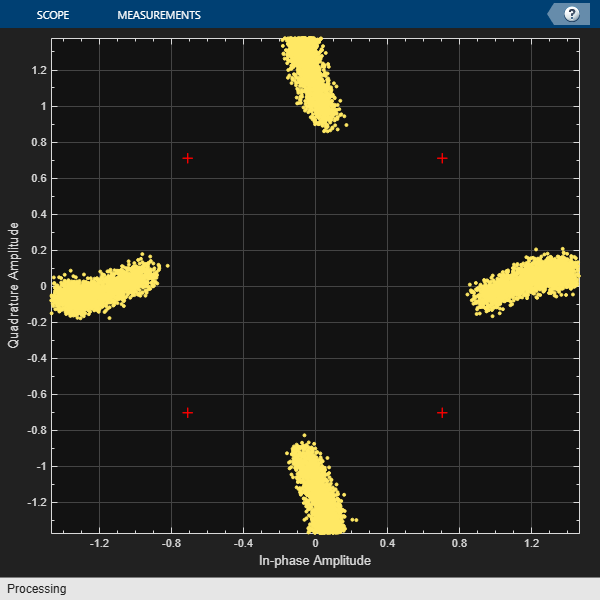The QPSK constellation shows the effects of the fading channel; however, the signal still has four identifiable states.

QPSK Modulation in Frequency-Selective Fading

Increase the symbol rate to 500 kbps and update the related channel property. Pass the signal through the Rayleigh channel and apply AWGN.

```symbolRate = 500e3; release(channel) channel.SampleRate = symbolRate; rxSig = awgn(channel(modSig),25); ```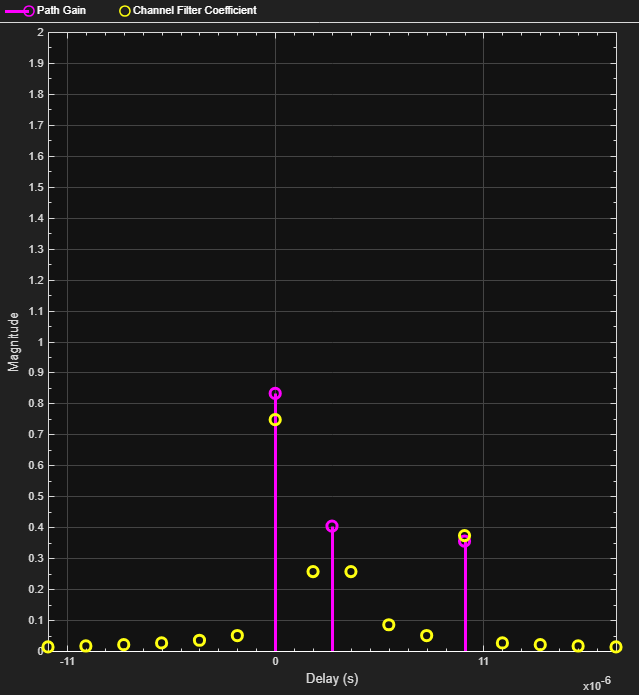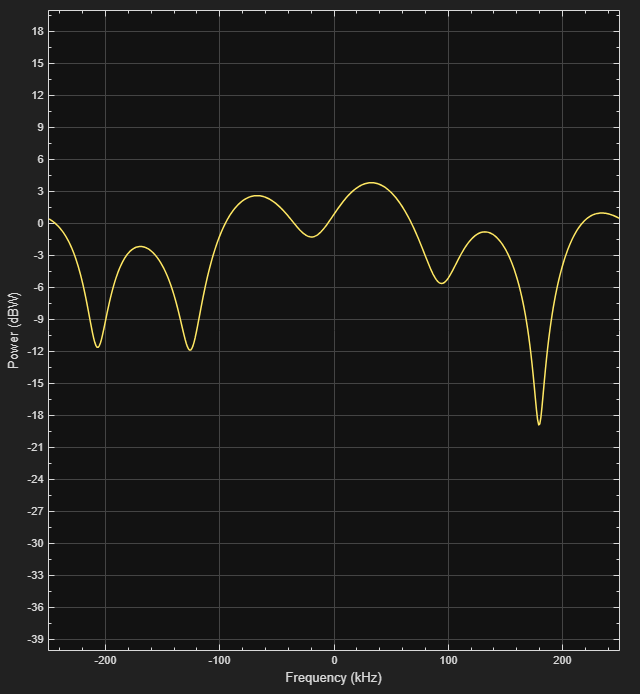The impulse and frequency responses show that the multipath fading is frequency selective.

Plot the constellation.

```constDiagram(rxSig) ```As the signal bandwidth is increased from 500 Hz to 500 kHz, the signal becomes highly distorted. This distortion is due to the intersymbol interference (ISI) that comes from time dispersion of the wideband signal. The delay span of the channel (10 microseconds) is now larger than the QPSK symbol period (2 microseconds) so the resultant bandlimited impulse response is no longer flat. Alternatively, the signal bandwidth is much larger than the coherence bandwidth, 50 kHz.

## Support

#### Bridging Wireless Communications Design and Testing with MATLAB

Download white paper